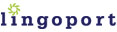# Locale-Sensitive Java Constructor

### `java.lang.Float`

``` Float(double value) Float(float value) Float(String s)```

### Internationalization (I18n) Method Overview

The `Float` class wraps a `float` primitive type in an object. To construct a `Float` object, you pass in either a `double` value, a `float` value, or a floating point string.

### I18n Issues

The problem with calling the `Float` constructor with a string parameter is that it is not locale aware. For example, if a string is set to `"2,3"` where a comma is used as the decimal separator, the `Float` constructor will not create the correct `Float` object. This is because it is designed to assume that a period will be used as the decimal separator.

### Suggested Replacement

To ensure that locale is applied when converting between float values and float strings, use the `DecimalFormat` class.

```Float myFloat = new Float(myFloatString); ```

Use:

``` Float myFloat = null; //Retrieve the runtime user's locale Locale locale = getUserLocale(); //Now call the NumberFormat factory method //and pass the locale object NumberFormat f = NumberFormat.getInstance(locale); //If it is in fact an instance of DecimalFormat, //cast it as such and use it as needed if (f instanceof DecimalFormat) {     try {         myFloat = new Float(((DecimalFormat) f).parse(myFloatString).floatValue());     } catch (ParseException e) {} } ```